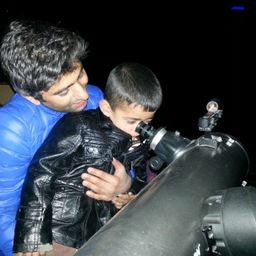Gravitation

### Discussion

You must be signed in to discuss.
##### Top Physics 101 Mechanics Educators##### Andy C.

University of Michigan - Ann Arbor##### Marshall S.

University of Washington##### Jared E.

University of Winnipeg

Lectures

Join Bootcamp

### Video Transcript

Let's write on the Cubans. We have ah, speed of spaceship. We is equal to 0.9 00 times the speed of light and, uh ah, spaceships landing major by a person on earth it is ever l is equal to 7 to 5 point zero meters. Uh, let's hold Bart A. We need to find out spaceships, Proper length, proper land. We know that l is equal to proper Linz Times square root off one minus B squared, divided by C square Now solving for proper land proper lend is equal to l divided by scared Toto one minus we squared, divided by six square. Now let's plug in the values proper length is equal to 75 divided by scared it off one minus. So we have a 0.900 square C squared gets canceled with C square and therefore proper land is equal to 1 72 point 02 meters. So properly and physical to 1 72 point 02 meters. Now let's do part B in barbecue, we need to find a time we know that s equals. We multiply every T and S in this case is, uh 1 72.2 meters and speed is 0.900 times the speed of light. Multiply the tea soling 40 t equals 1 72.2 divided by 0.9 toodles. You don't want to lie by three. Multiply by 10 to the power eight and this gives this five point zero tree multiplied by 10 to the power minus seven seconds and bark. See again using accessible to the multiplier E t. And it's now in this case is 75 meters. Um 75 is equal to speed is the same 0.900 times the speed of light. Want to live a t in solving for t T equals 75 divided by 0.900 times Ah, speed of light and dividing. We have d equals dew 0.19 Multiply by 10 to the power minus eight seconds#### Topics

Gravitation

##### Top Physics 101 Mechanics Educators##### Andy C.

University of Michigan - Ann Arbor##### Marshall S.

University of Washington##### Jared E.

University of Winnipeg

Lectures

Join Bootcamp